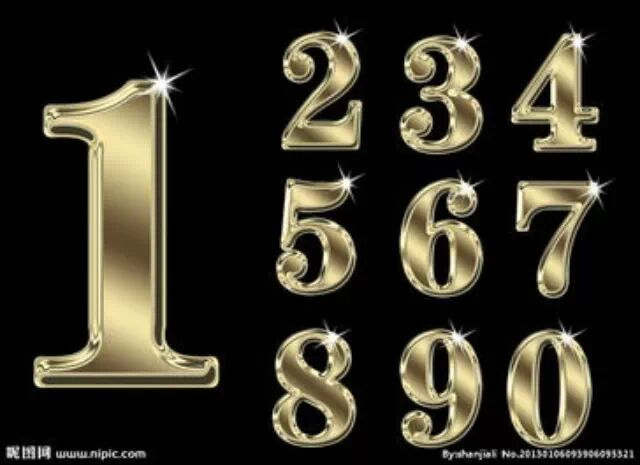Page Views :smartshare150403a瀏覽次數：1.十几乘十几：

2+4=6
2x4=8
12×14=168

２.头相同，尾互补(尾相加等于10)：

2x3=6
3x7=21
23×27=621

３.第一个乘数互补，另一个乘数数字相同：

4×4=16
7×4=28
37×44=1628

４.几十一乘几十一：

2+4=6
1×1=1
21×41=861

５.11乘任意数：

3+1=4
1+2=3
2+5=7
2和5分别在首尾
11×23125=254375

６.十几乘任意数：

3×3+2=11
3×2+6=12
3×6=18
13×326=4238*WIO - Walk In OnlineWalk In Online International Group  沃盈网联商城国际集团 China, Hong Kong, Malaysia, Singapore, Indonesia, Thailand 中国, 香港, 马来西亚, 新加坡, 印尼, 泰国 Email : hq@walkinonline.com